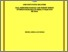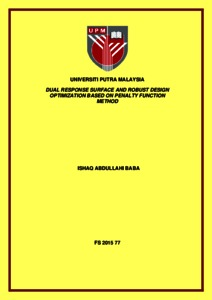# Dual response surface and robust design optimization based on penalty function method

## Citation

Baba, Ishaq Abdullahi (2015) Dual response surface and robust design optimization based on penalty function method. Masters thesis, Universiti Putra Malaysia.

## Abstract

Despite all the existing optimization schemes for solving dual response surface problem, the tradeoffs between the mean and variance functions remain unsolved. It is now evident that selecting an appropriate optimization scheme in the determination of the optimal setting conditions is critical. Most of the existing optimization schemes do not take into account the measure on violation of constraint in the conversion of the constrained to an unconstrained optimization. The purpose of this thesis is to introduce a new optimization scheme based on the penalty function method. The penalty function method converts the constrained into unconstrained optimization problem by adding the constraint to the original objective function. The advantage of the new approach is that it takes into consideration the constraint by introducing a penalty constant. The performance of the new proposed technique is compared with three other existing techniques Dual response surface optimization uses the ordinary least squares method OLS to determine the adequate process mean and variance response functions by assuming that the design data come from a normal distribution function and there is no outlier in the data set. Under this condition, the sample mean and sample variance are the most appropriate method to estimate the mean and variance of the response variables. However, the sample mean and the sample variance are duly affected by outlier in which may lead to producing inconsistent estimates of coefficient of the regression and sometime even change the sign of regression line. Robust methods are design to remedy this type of problems. Median and median absolute deviation MAD are robust substitute of mean and variance of response variables respectively. Nonetheless, they are known to be less efficient than mean in uncontaminated data. It is part of our objective in this thesis, to propose using a highly efficient and resistant robust location and scale of the MM estimator for estimating the mean and variance of the response variables using the new proposed optimization scheme and also to propose MM estimator to estimate the parameters of response function for the process mean and variance. Furthermore, the usual assumption of equal number of replications at each design point during an experiment is often impractical in real life industrial application of dual robust design optimization. In this case, applying the ordinary least squares method, OLS to determine the estimated response functions for mean and variance processes may be affected by the presence of heteroscedasticity problem. As our third objective in this thesis, we proposed using the weighted least squares method, WLS to estimate the parameters of the regression model and apply the new optimization scheme to find the optimal setting conditions for the estimation of the optimal mean response. The overall results signify that the proposed optimization scheme PM is superior to other existing optimization schemes. The PM based on the proposed MM robust location and scale estimator also outperforms other methods. Finally, the proposed method based on WLS turned out to be the most efficient method in the presence of heteroscedasticity problem.Preview
Text
FS 2015 77 IR.pdfView Item